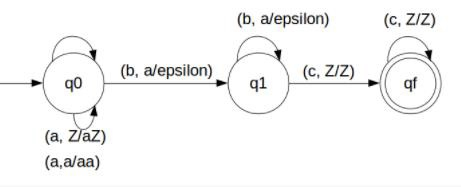# Construct DPDA for anbncm where, n,m≥1 in TOC

DPDA is the short form for the deterministic push down automata (DPDA).

## Problem

Construct DPDA for anbncm where m,n>=1

## Solution

So, the strings which are generated by the given language are −

L={abc,aabbc,aaabbbcc,….}

That is we have to count equal number of a’s, b’s and different number of c’s

Let’s count the number of a's which is equal to the number of b's.

This can be achieved by pushing a's in STACK and then we will pop a's whenever "b" comes.

Then for c nothing will happen.

Finally, at the end of the strings if nothing is left in the STACK then we can say that language is accepted in the PDA.

The PDA for the given problem is as follows −## Transition Function

The transition functions are as follows −

Step 1 − δ(q0, a, Z) = (q0, aZ)

Step 2 − δ(q0, a, a) = (q0, aa)

Step 3 − δ(q0, b, a) = (q1, ε)

Step 4 − δ(q1, b, a) = (q1, ε)

Step 5 − δ(q1, c, Z) = (qf, Z)

Step 6 − δ(qf, c, Z) = (qf, Z)

## Explanation

Step 1 − Consider an input string: "aabbcc" which satisfies the given condition.

Step 2 − Scan string from left to right.

Step 3 − First input is 'a' and the rule:

for input 'a' and STACK alphabet Z, then

push the input 'a' into STACK: (a,Z/aZ) and state will be q0

Step 4 − Second input is 'a' and the rule:

for input 'a' and STACK alphabet 'a', then

push the input 'a' into STACK : (a,a/aa) and state will be q0

Step 5 − Third input is 'b' and the rule:

for input 'b' and STACK alphabet 'a', then

pop STACK with one 'a' : (b,a/&epsiloon;) and state will be now q1

Step 6 − Fourth input is 'b' and the rule −

For input 'b' and STACK alphabet 'a' and state is q1, then

pop STACK with one 'a' : (b,a/&epsiloon;) and state will be q1

Step 7 − Fifth input is 'c' and top of STACK is Z the rule:

for input 'c' and STACK alphabet 'Z' and state is q1, then

do nothing: (c,Z/Z) and state will be qf

Step 8 − Sixth input is 'c' and top of STACK is Z the rule:

for input 'c' and STACK alphabet 'Z' and state is qf, then

do nothing: (c,Z/Z) and state will be qf

Step 9 − We reached end of the string, and the rule:

If we are standing at final state, qf then string is accepted in the PDA